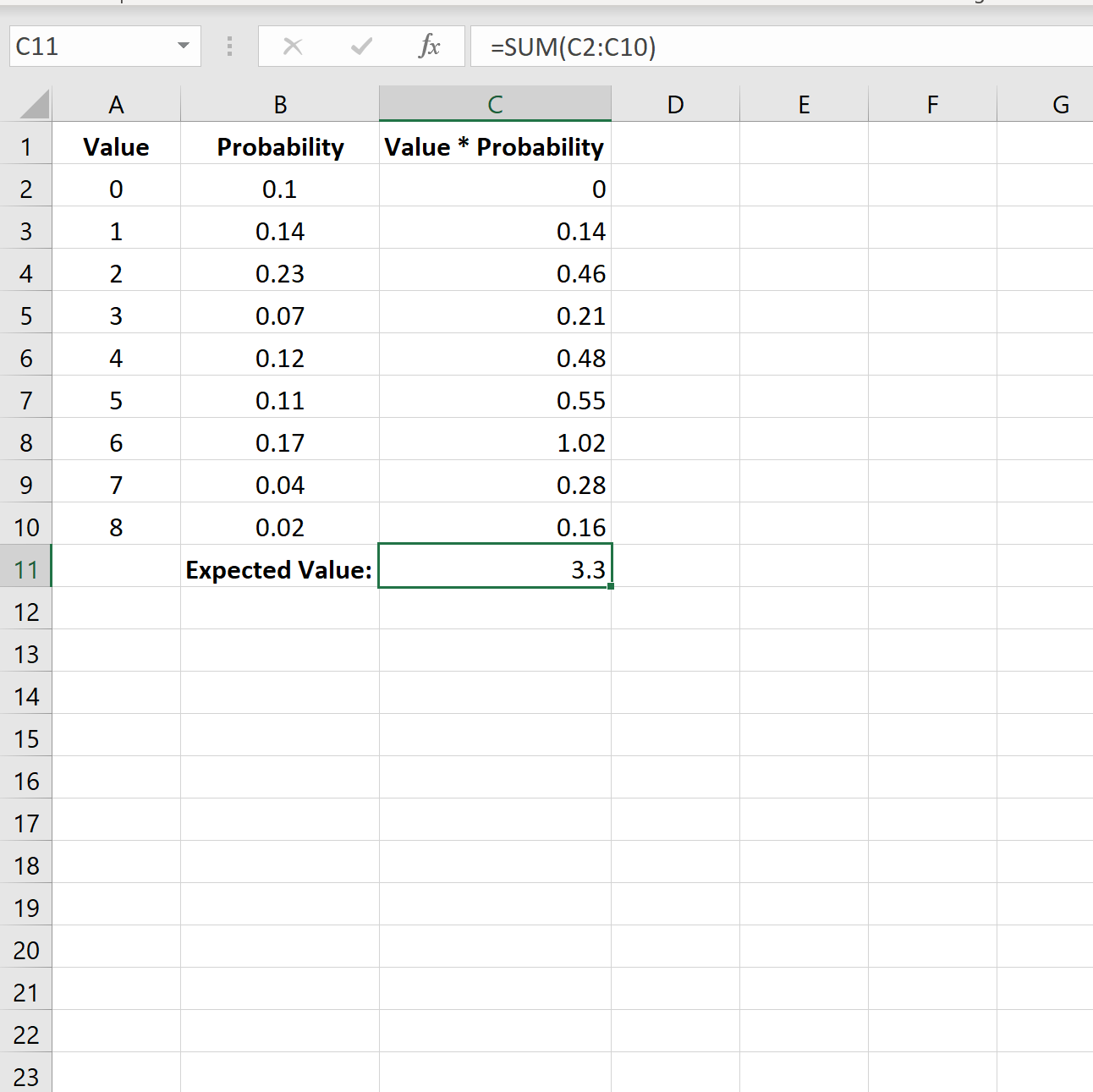Blog

# How to Find Expected Value in Excel?

Are you looking to find expected value in Excel but don’t know where to start? You’ve come to the right place! Knowing how to find expected value in Excel can be incredibly useful for anyone working with data. Whether you’re a student, a professional, or an Excel enthusiast, this article will guide you through the expected value calculation process step by step. By the end of this article, you will have a better understanding of expected value and be able to calculate it quickly and accurately using Excel. Let’s get started!### What is the expected value in Excel?

The expected value in Excel is a statistical measure of a probability distribution that calculates the average outcome when the same experiment is repeated multiple times. It is used to determine the average result of a particular investment or event. It is also known as the mathematical expectation, mean, or average.

### How is expected value calculated in Excel?

Expected value in Excel is calculated by multiplying the probability of each outcome by its value, and then summing up all these products. The formula for calculating expected value in Excel is EV = Σ (probability x outcome).

### What is an example of expected value in Excel?

An example of expected value in Excel is calculating the expected return on an investment. For example, if an investor has a 75% chance of making a 10% return and a 25% chance of making a 5% return, the expected value of the return would be (0.75 x 0.1) + (0.25 x 0.05) = 0.0875 or 8.75%.

### How can expected value be used in Excel?

Expected value can be used in Excel to assess the average outcome of an investment or event. It can also be used to identify the most likely outcome of a particular situation. This can be useful for financial planning and decision making.

### What are the benefits of using expected value in Excel?

Using expected value in Excel can help investors and decision makers make informed decisions. It can help them identify the most likely outcome of a particular situation and assess the expected return of an investment. It can also be used to compare different scenarios and calculate the expected value of each one.

### What are the limitations of using expected value in Excel?

The limitations of using expected value in Excel include the fact that it does not take into account variables that are unknown or difficult to quantify, such as human behavior or external events. Additionally, expected value does not guarantee that the expected outcome will actually occur. It is simply an estimate of the average outcome.

The expected value of a random variable is a powerful tool for making decisions, and Excel makes it easy to calculate. With the right formulas and a bit of knowledge of how Excel works, you can quickly and accurately find the expected value of any random variable. Knowing how to calculate expected value in Excel can help you make informed decisions and maximize your potential return.